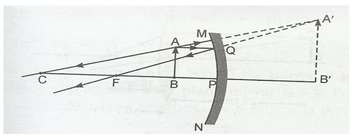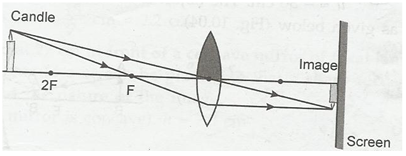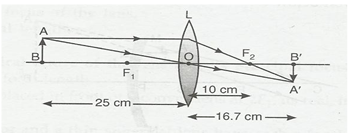### Light Reflection and Refraction - Solutions

CBSE Class 10 Science

NCERT Solutions
Chapter -  10
Light: Reflection and Refraction

Page No. 168

1. Define the principle focus of a concave mirror.

Ans. Principal focus of a concave mirror is the point on its principal axis, where light rays coming parallel to principal axis actually converge after reflection from mirror.

2. The radius of curvature of a spherical mirror is 20 cm. what is its focal length?

Ans. Focal length (f)= R/2 = 20 cm/2 = 10 cm.

3. Name a mirror that can give an erect and enlarged image of an object.

Ans. Only a concave mirror can give a erect and enlarged image of an object.

4. Why do we prefer a convex mirror as a rear-view mirror in vehicles?

Ans. We prefer a convex mirror as a rear-view mirror in vehicles because a convex mirror gives an erect and diminished image. As a result, convex mirror help the driver to have much wider field view.

Page No. 171

1. Find the focal length of a convex mirror whose radius of curvature is 32 cm.

Ans. Radius of curvature of (R) = 32 cm

Focal length(f) = R/2 = 32/2 cm = 16 cm.

2. A concave mirror produces three times magnified real image of an object placed at 10 cm in front of it. Where is the image located?

Ans. Distance of object from concave mirror (u)= -10 cm.

Magnification (m) = -3

m = -v/u

v = -mu = -(3) x (-10) = -30 cm.

Page No. 176

1. A ray of light traveling in air enters obliquely into water. Does the light ray bend towards or away from the normal? Why?

Ans. The light bends towards the normal on entry into water. It is due to the fact that as compared to air, the water is optically denser medium.

2. Light enters from air to glass having refractive index 1.50. What is the speed of light in glass? The speed of light in vacuum is 3x108 m/s.

Ans. Speed of light in vacuum (c) = 3 x 108 m/s.

Refractive index = c/v.

Speed of light in glass = 3 x 108 m/s/ 1.50

= 2 x 108 m/s

3. Find out, from Table (10.3), the medium having highest optical density. Also, find the medium with lowest optical density.

Ans. As per table, diamond has highest optical density (2.42). Medium with lowest optical density is air (1.0003)

4. You are given kerosene, turpentine and water. In which of these does the light travel fastest? Use the information given in table 10.3

Ans. As the refractive index of water is least out of three substances, hence speed of light is maximum in water. So, light travels fastest in water.

5. The refractive index of diamond is 2.42. What is the meaning of this statement?

Ans. It means that speed of light in diamond is 2.42 times slower than speed of light in air.

Page No. 184

1. Define 1 dioptre of power of lens.

Ans. One dioptre of is defined as the power of lens having a focal length of 1 m.

2. A convex lens forms a real and inverted image of a needle at distance of 50 cm. from it. Where is the needle placed in front of the convex lens if the image is equal to the size of objects? Also, find the power of lens.

Ans. Image distance (v) = +50 cm, h= ho

hi/ho = v/u

u = v x h/ hi

= 50 x h/ hi

= 50 cm.

Now,

u = -50 cm

v = + 50 cm.

f = ?

1/f = 1/v – 1/u

1/f = 1/50 + 1/50

f = + 25 cm. = 0.25 m

Power of lens (P) = 1/f

= 1/ 0.25 = + 4D.

3. Find the power of a concave lens of focal length 2 m.

Ans. Focal length of concave lens = - 2 m.

P = 1/f = 1/ (-2m)

= -0.5 D

TEXTBOOK EXERCISE

1. Which one of the following materials cannot be used to make a lens?

(a) Water

(b) Glass

(c) Plastic

(d) Clay

Ans. (d) Clay

2. The image formed by a concave mirror is observed to be virtual, erect and larger than the object. Where should be the position of the object?

(a) Between the principal focus and the centre of curvature.

(b) At the centre of curvature

(c) Beyond the centre of curvature

(d) Between the pole of the mirror and its principal focus.

Ans. (d) Between the pole of the mirror and its principal focus.

3. Where should an object be placed in front of convex lens to get a real image of the size of the object?

(a) At the principal focus of the lens.

(b) At twice the focal length

(c) At infinity

(d) Between the optical centre of the lens and its principal focus.

Ans. (b) At twice the focal length ( centre of curvature)

4. A spherical mirror and thin spherical lens have each of focal length of -15 cm. the mirror and lens are likely to be

(a) Both concave

(b) Both convex

(c) The mirror is concave and the lens is convex

(d) The mirror is convex and lens is concave.

Ans. (a) both concave.

5. No matter how far you stand from a mirror, your image appears erect. The mirror is likely to be

(a) Plane

(b) Concave

(c) Convex

(d) Either concave or convex.

Ans. (d) either plane or convex

6. Which of the following lens would you prefer to use while reading small letters found in a dictionary?

(a) A convex lens of focal length 50 cm

(b) A concave lens of focal length 50 cm

(c) A convex lens of focal length 5 cm

(d) A concave lens of focal length 5 cm

Ans. (c) A convex lens of focal length 5 cm.

7. We wish to obtain an erect image of an object, using a concave mirror of focal length 15 cm. What should be the range of distance of the object from mirror? What is the nature of image? Is the image larger or smaller than the object? Draw a ray diagram to show the image formation in this case.

Ans. Object must be placed in front of concave mirror between its pole and principal focus at a distance less than 15 cm.

The image formed will be virtual and erect. The size of the image is larger the object. The ray diagram is as follows:8. Name the type of mirror used in the following situations:

(b) Side/rear-view mirror of a vehicle.

(c) Solar furnace.

Ans. (a) Headlights of a car- concave mirror to give parallel beam of light after reflection from concave mirror.

(b) Side/rear-view mirror of vehicle- convex mirror as it forms virtual erect and diminished image to give wider view field.

(c) Solar furnace- concave mirror to concentrate sunlight to produce heat in solar furnace.

9. One-half of a convex lens is covered with a black paper. Will this lens produce a complete image of the object? Verify your answers experimentally. Explain your observations.

Ans. When one-half of a convex lens is covered with a black paper, this lens produces a complete image of the object. To prove it we perform experiment:Take a concave mirror and cover half part of its by using black paper. Place it vertically in a stand. On one side of it place a burning candle. On opposite side of the lens fix a white screen. Adjust the position of candle or screen till clear image of burning candle is formed on the screen. We observe that the image is complete image of the object.

From the experimental observations, we find that image formation does not depend upon the size of a lens. A similar lens can also form complete image of an object placed in front of it. However, brightness of the image decreases when some part of lens is blocked. It is because now lesser number of rays pass through the lens.

10. An object 5 cm in length is held 25 cm away from a converging lens of focal length 10 cm. Draw the ray diagram and find the position, size and the nature of the image formed.

Ans. f= +10 cm, u= -25 cm and ho= 5 cm1/f = 1/v – 1/u

1/v = 1/10 -1/15

1/v = 3/50

V = 50/3 cm.

The image is real and inverted at a distance of 16.7 cm from the lens on opposite side.

Magnification (m) = hi/h0= v/u

hi /5 =16.7/-25

hi = -10/3 cm. image is inverted and diminished.